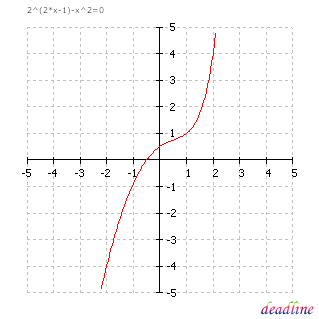﻿ Solve exponential equations: 2^(2*x-1)-x^2 = 0

# Solve exponential equations

Problem: solve an exponential equation

Equation to solve: 2^(2*x-1)-x^2 = 0

Interval for roots: [-5 ; 5]

DeadLine can solve exponential equations instantly, providing the equation graph and the roots.

Number of roots found: 1

x1 = -0,5

Tip: the graph produced by DeadLine indicates that the exponential function is monotonic and the corresponding equation has an unique root.